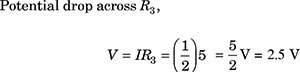﻿ Board Paper of Class 12 2022 Physics-G12 Term-1 Set-2 Solutions | Aasoka

# Board Paper of Class 12 2022 Physics-G12 Delhi Set 55/2/4 - Solutions

#### General Instructions:

1. This question paper contains 55 questions out of which 45 questions are to be attempted. All questions carry equal marks.
2. This question paper consists of three sections , Section A, Section B and Section C.
3. Section A contains 25 questions. Attempt any 20 questions from Q. No. 1 to 25.
4. Section B contains 24 questions. Attempt any 20 questions from Q. No. 26 to 49.
5. Section C contains 6 questions. Attempt any 5 questions from . No. 50 to 55.
6. The first 20 questions in Section A and Sections B and first 5 questions attempted in Section C by a candidate will be evaluated.
7. There is only one correct option for every multiple choice question (MCQ). Marks will not be awarded for answering more than one option.
8. There is no negative marking.

#### Section – A

This Section consists of 25 multiple choice questions with overall choice to attempt any 20 questions. In case more than desirable number of questions are attempted, only first 20 questions will be considered for evaluation.

## A negative charged object X is repelled by another charged object Y. However an object Z is attracted to object Y. Which of the following is the most possibility for the object Z?

1. positively charged only
2. negatively charged only
3. neutral or positively charged
4. neutral or negatively charged

Ans. (c)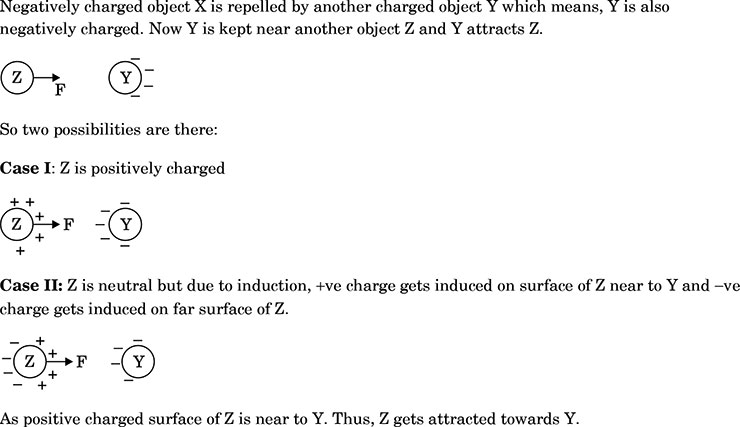## In an experiment three microscopic latex spheres are sprayed into a chamber and became charged with charges +3e, +5e and -3e respectively. All the three spheres came in contact simultaneously for a moment and got separated. Which one of the following are possible values for the final charge on spheres?

1. +5e, -4e, +5e
2. +6e, +6e, -7e
3. -4e, +3.5e, +5.5e
4. +5e, -8e, +7e

Ans. (b)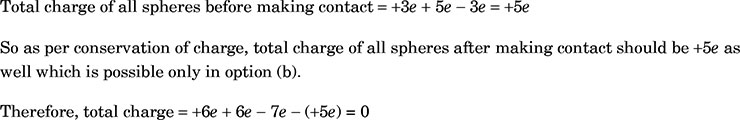## An object has charged of 1 C and gains $5.0×{10}^{18}$ electrons. The net charge on the object becomes-

1. -0.80 C
2. +0.80 C
3. +1.80 C
4. +0.20 C

Ans. (d)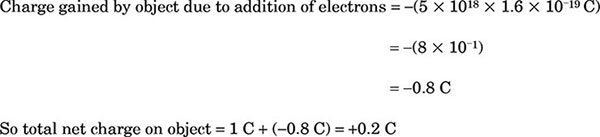## Kirchhoff’s first rule $\mathrm{\Sigma I}=0$ and second rule $\mathrm{\Sigma IR}=$ E (where the symbols have their usual meanings) are respectively based on–

1. conservation of momentum and conservation of charge
2. conservation of energy, conservation of charge
3. conservation of charge, conservation of momentum
4. conservation of charge, conservation of energy

Ans. (a)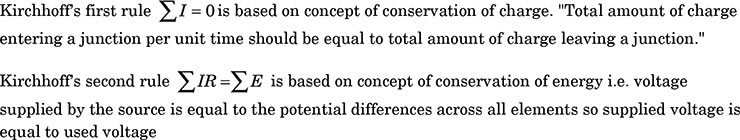## The electric power consumed by a 220 V - 100 W bulb when operated at 110 V is

1. 25 W
2. 30 W
3. 35 W
4. 45 W

Ans. (a)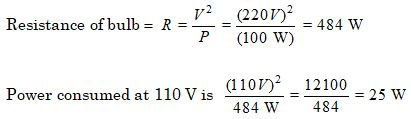## Which one of the following has negative temperature coefficient of resistivity?

1. metal
2. metal and semiconductor
3. semiconductor
4. metal and alloy

Ans. (c)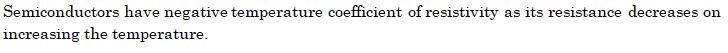## Two wires carrying currents ${\mathrm{I}}_{1}$, ${\mathrm{I}}_{2}$ lie, one slightly above the other, in a horizontal plane as shown in figure. The region of vertically upward strongest magnetic field is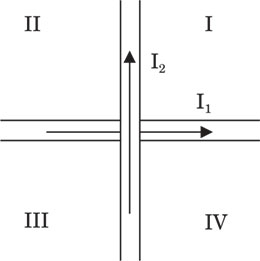1. I
2. II
3. III
4. IV

Ans. (b)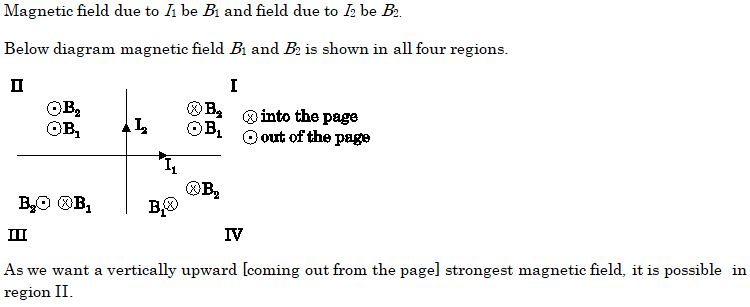## Two parallel conductors carrying current of 4.0 A and 10.0 A are placed 2.5 cm apart in vacuum. The force per unit length between them is-

Ans. (d)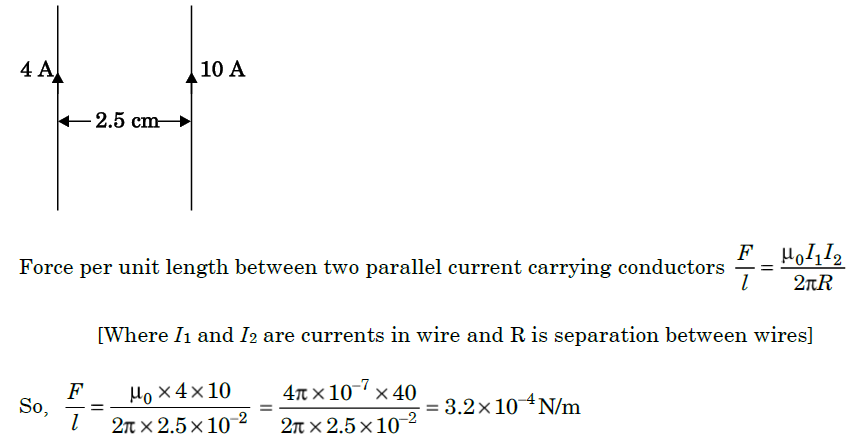## If an ammeter is to be used in place of a voltmeter, then we must connect with the ammeter a -

1. low resistance in parallel
2. low resistance in series
3. high resistance in parallel
4. high resistance in series

Ans. (d)

## The magnetic field at the centre of a current carrying circular loop of radius R, is ${\mathrm{B}}_{1}$. The magnetic field at a point on its axis at distance R from the center of the loop ${\mathrm{B}}_{2}$. Then the ratio (${\mathrm{B}}_{1}$/${\mathrm{B}}_{2}$) is

1. $2\sqrt{2}$
2. $\frac{1}{\sqrt{2}}$
3. $\sqrt{2}$
4. 2

Ans. (a)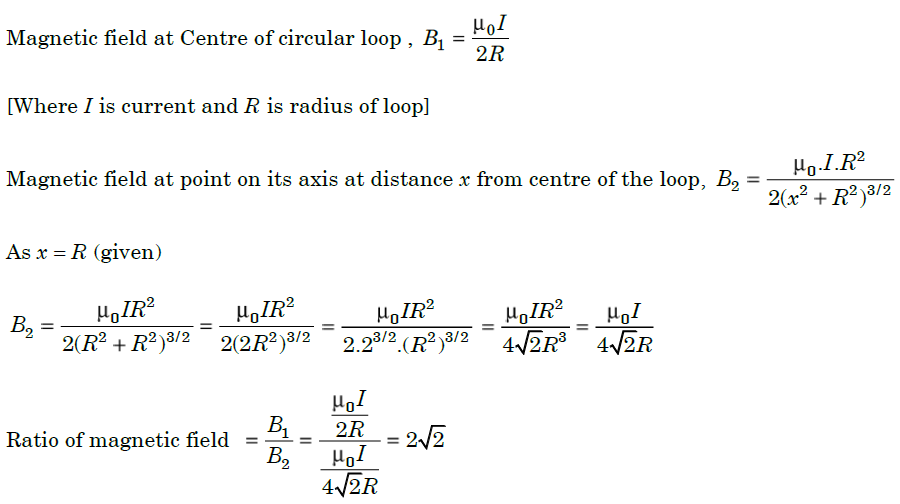## The self-inductance of a solenoid of 600 turns is 108 mH. The self-inductance of a coil having 500 turns with the same length, the same radius and the same medium will be

1. 95 mH
2. 90 mH
3. 85 mH
4. 75 mH

Ans. (d)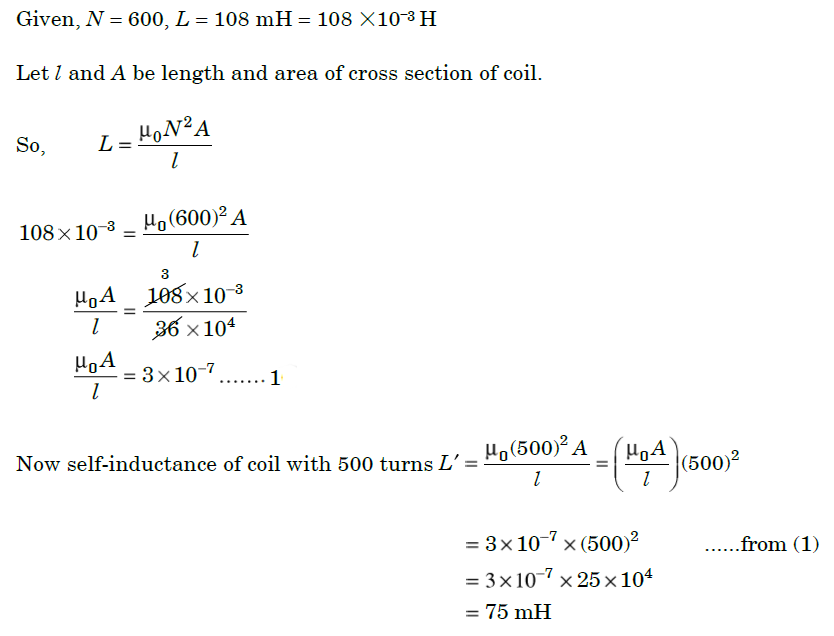## The rms current in a circuit connected to a 50 Hz AC source is 15 A. The value of the current in the circuit $\left(\frac{1}{600}\right)$ s after the instant the current is zero, is -

1. $\frac{\mathbf{15}}{\sqrt{\mathbf{2}}}\mathrm{A}$
2. 8 A

Ans. (a)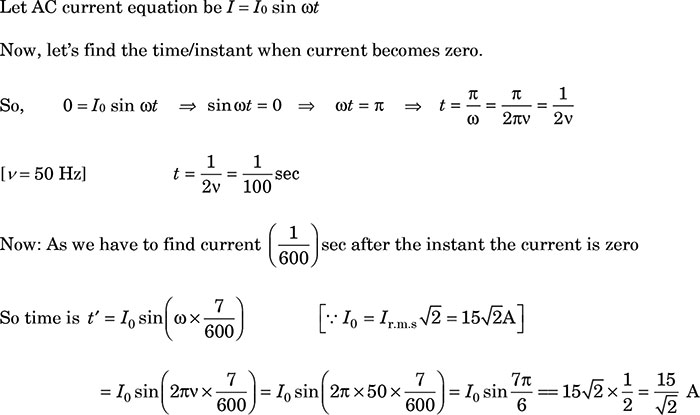## In a circuit the phase difference between the alternating current and the source voltage is $\frac{\mathrm{\pi }}{2}$. Which of the following cannot be the element(s) of the circuit?

1. only C
2. only L
3. L and R
4. L or C

Ans. (c)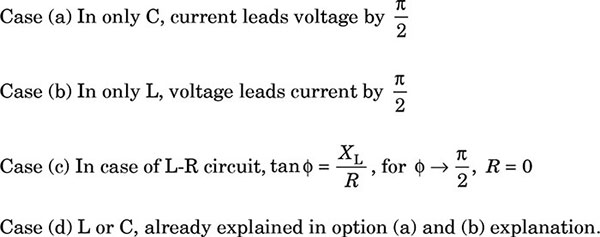## The electric potential V at any point (x, y, z) is given by V = $3{\mathrm{x}}^{2}$ where x is in meters and V in volts. The electric field at the point (1 m, 0, 2m) is –

1. 6 V/m along -x-axis
2. 6 V/m along +x-axis
3. 1.5 V/m along -x-axis
4. 1.5 V/m along +x-axis

Ans. (a)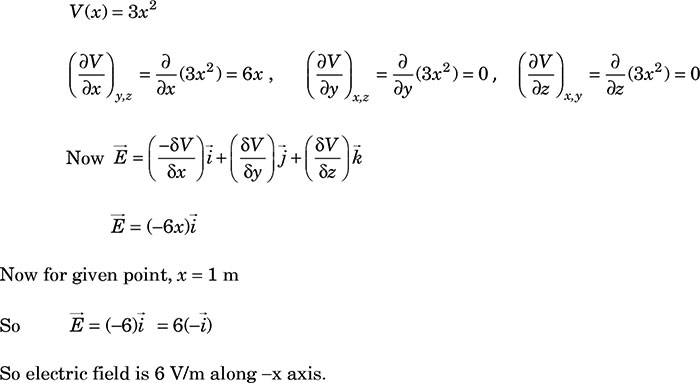## Which of the diagrams correctly represents the electric field between two charged plates if a neutral conductor is placed in between the plates?

1.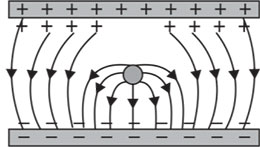2.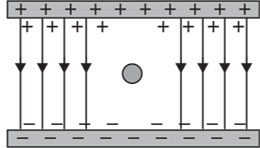3.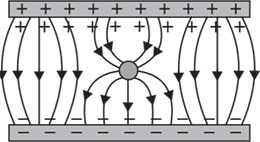4.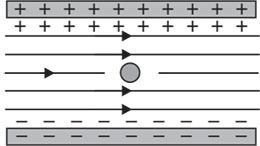Ans. (d)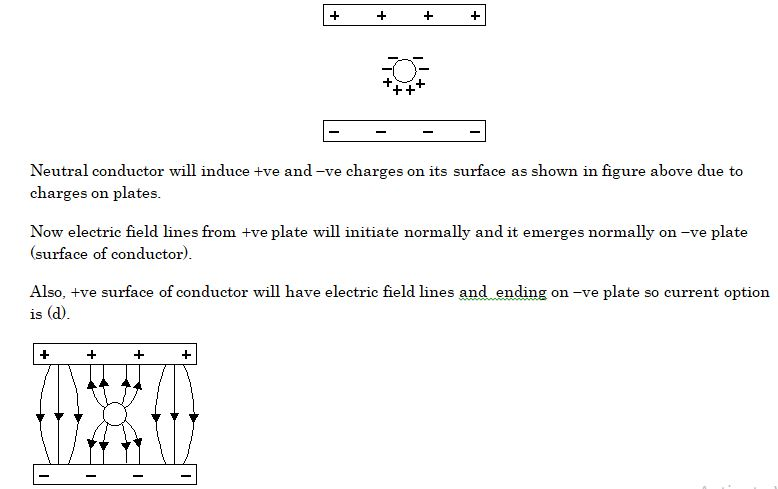## A variable capacitor is connected to a 200 V battery. If its capacitance is changed from 2 $\text{μF}$ to x$\text{μF}$, the decrease in energy of the capacitor is 2 × ${10}^{-2}$ J. The value of x is -

1. 1 $\text{μF}$
2. 2 $\text{μF}$
3. 3 $\text{μF}$
4. 4 $\text{μF}$

Ans. (a)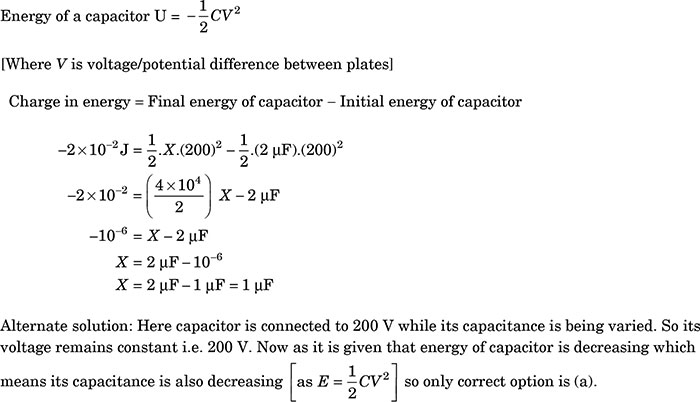## A potential difference of 200 V is maintained across a conductor of resistance 100 $\mathrm{\text{Ω}}$. The number of electrons passing through it in 1s is

Ans. (a)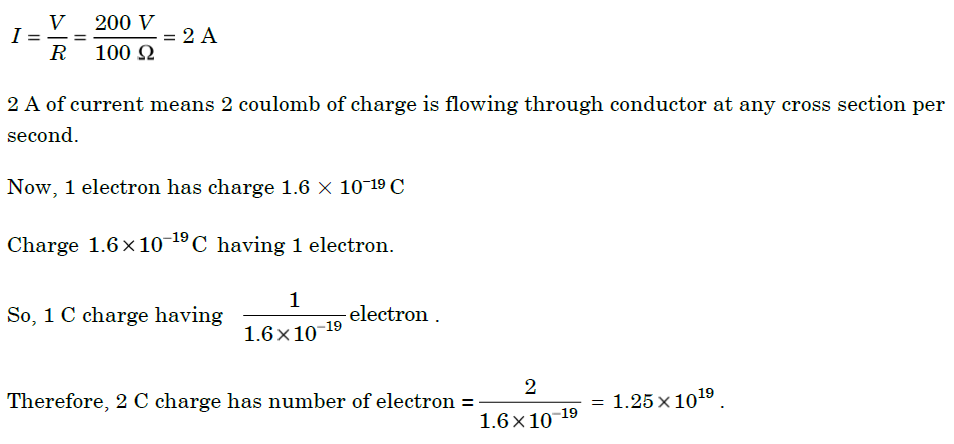## The impedance of a series LCR circuit is -

1. $\sqrt{\frac{1}{{\mathrm{X}}_{\mathrm{C}}^{2}}+\frac{1}{{\mathrm{X}}_{\mathrm{L}}^{2}}+{\mathrm{R}}^{2}}$
2. $\sqrt{{\mathrm{R}}^{2}+{\left({\mathrm{X}}_{\mathrm{L}}-{\mathrm{X}}_{\mathrm{C}}\right)}^{2}}$

Ans. (d)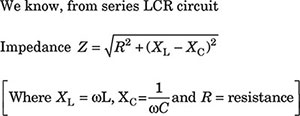## When an alternating voltage E = ${\mathrm{E}}_{\mathrm{0}}$ sin $\mathrm{\omega }$t is applied to a circuit, a current I = ${\mathrm{I}}_{\mathrm{0}}$ sin flows through it. The average power dissipated in the circuit is

1. Erms . Irms
2. E0 I0
3. Zero

Ans. (d)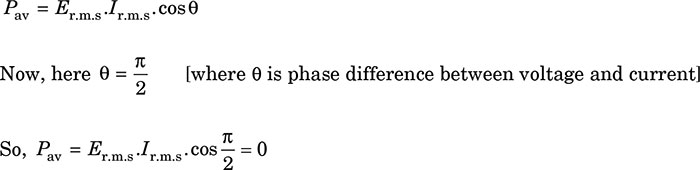## A current carrying wire kept in a uniform magnetic field, will experience a maximum force when it is

1. perpendicular to the magnetic field
2. parallel to the magnetic field
3. at an angle of 45° to the magnetic field
4. at an angle of 60° to the magnetic field

Ans. (a)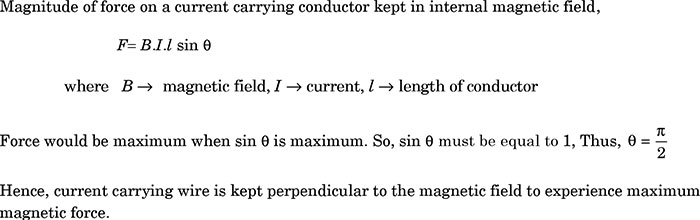1. 5 V
2. 20 V
3. 25 V
4. 65 V

Ans. (c)

## In a DC circuit the direction of current inside the battery and outside the battery respectively are –

1. positive to negative terminal and negative to positive terminal
2. positive to negative terminal and positive to negative terminal
3. negative to positive terminal and positive to negative terminal
4. negative to positive terminal and negative to positive terminal

Ans. (c)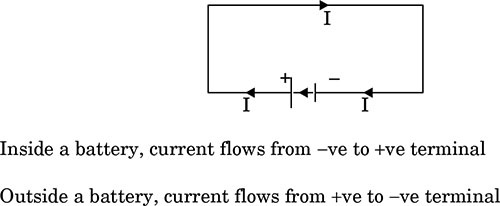## The magnitude of electric field due to a point charge 2q, at distance r is E. Then the magnitude of electric field due to a uniformly charged thin spherical shell of radius R with total charge q at a distance

1. $\frac{\mathrm{E}}{4}$
2. 0
3. 2E
4. 4E

Ans. (c)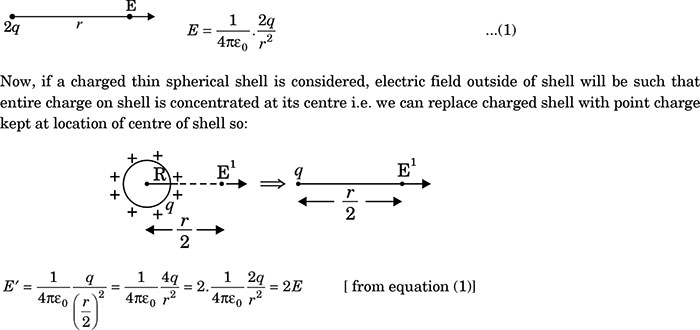## The horizontal component of earth’s magnetic field at a place is 0.2 G whereas it’s total magnetic field is 0.4 G. The angle of dip at the place is

1. 30°
2. 45°
3. 60°
4. 90°

Ans. (c)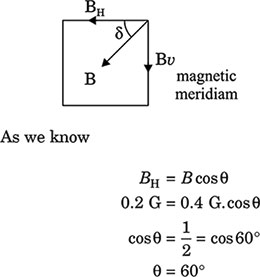## The current in the primary coil of a pair of coils changes from 7 A to 3 A in 0.04 s. The mutual inductance between the two coils is 0.5 H. The induced emf in the secondary coil is –

1. 50 V
2. 75 V
3. 100 V
4. 220 V

Ans. (a)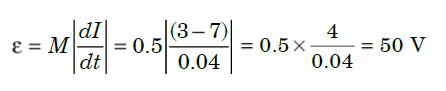## A square sheet of side ‘a’ is lying parallel to XY plane at z = a. The electric field in the region is . The electric flux through the sheet is

1. a4c
2. $\frac{1}{3}{\mathrm{a}}^{3}\mathrm{c}$
3. $\frac{1}{3}{\mathrm{a}}^{4}\mathrm{c}$
4. 0

Ans. (a)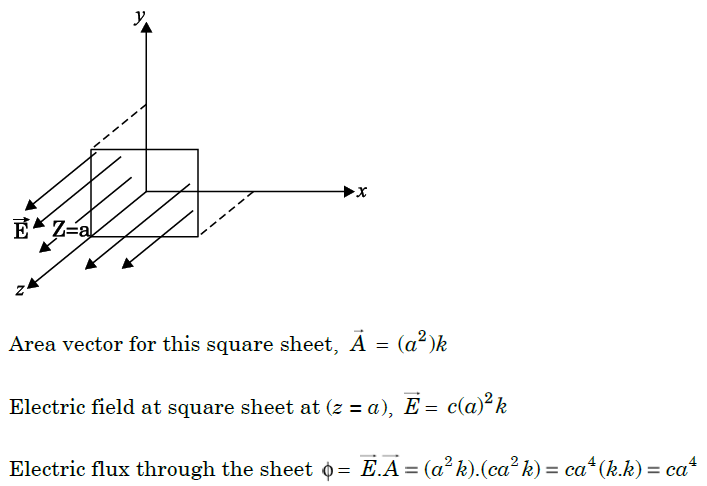## Three charges q, –q and q0 are placed as shown in figure. The magnitude of the net force on the charge q0 at point O is $\left[K=\frac{1}{\left(4\pi \right){\in }_{0}}\right]$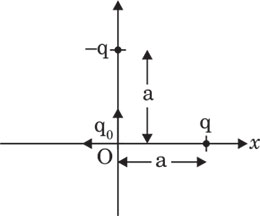1. 0
2. $\frac{2{\mathrm{Kqq}}_{0}}{{\mathrm{a}}^{2}}$
3. $\frac{\sqrt{2}{\mathrm{Kqq}}_{0}}{{\mathrm{a}}^{2}}$
4. $\frac{1}{\sqrt{2}}\frac{{\mathrm{Kqq}}_{0}}{{\mathrm{a}}^{2}}$

Ans. (c)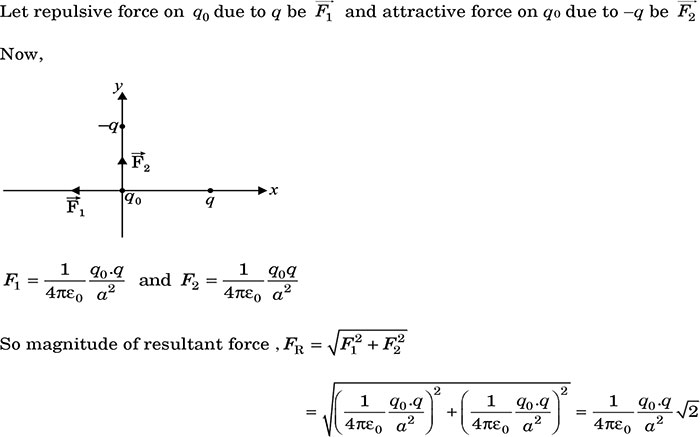## A + 3.0 nC charge Q is initially at rest at a distance of r1 = 10 cm from a + 5.0 nC charge q fixed at the origin. The charge Q is moved away from q to a new position at r2 = 15 cm. In this process work done by the field is

1. 1.29 x 10-5 J
2. 3.6 x 105 J
3. -4.5 x 10-7 J
4. 4.5 x 10-7 J

Ans. (d)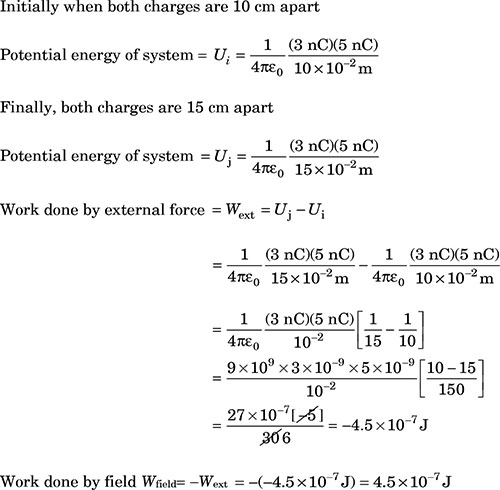## A car battery is charged by a 12 V supply, and energy stored in it is 7.20 x 105 J. The charge passed through the battery is –

1. 6.0 x 104 C
2. 5.8 x 103 J
3. 8.64 x 106 J
4. 1.6 x 106 C

Ans. (a)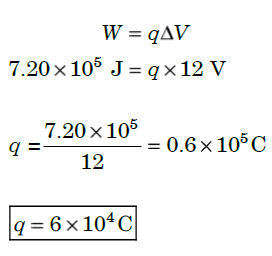## A straight conducting rod of length l and mass m is suspended in a horizontal plane by a pair of flexible strings in a magnetic field of magnitude B. To remove the tension in the supporting strings, the magnitude of the current in the wire is

1. $\frac{\mathrm{mgB}}{l}$
2. $\frac{\mathrm{mg}l}{\mathrm{B}}$

Ans. (c)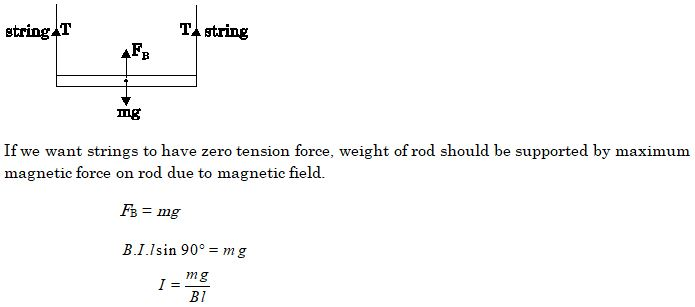## A constant current is flowing through a solenoid. An iron rod is inserted in the solenoid along its axis. Which of the following quantities will not increase?

1. The magnetic field at the centre
2. The magnetic flux linked with the solenoid
3. The rate of heating
4. The self-inductance of the solenoid

Ans. (c)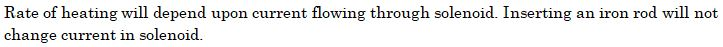## A circuit is connected to an AC source of variable frequency. As the frequency of the source is increased, the current first increases and then decreases. Which of the following combinations of elements is likely to comprise the circuit?

1. L, C and R
2. L and C
3. L and R
4. R and C

Ans. (a)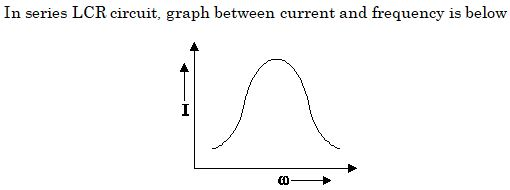## If n, e,$\tau$ and m have their usual meanings, then the resistance of a wire of length l and cross-sectional area A is given by -

Ans. (b)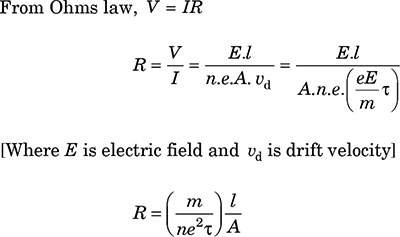## A proton and an alpha particle move in circular orbits in a uniform magnetic field. Their speeds are in the ratio of 9:4. The ratio of radii of their circular orbits $\left(\frac{{r}_{p}}{{r}_{\mathrm{alpha}}}\right)$ is

1. $\frac{3}{4}$
2. $\frac{4}{3}$
3. $\frac{8}{9}$
4. $\frac{9}{8}$

Ans. (d)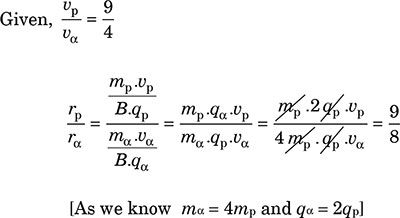## A coil of area 100 cm2 is kept at an angle of 30° with a magnetic field of 10-1 T. The magnetic field is reduced to zero in 10-4. The induced emf in the coil is -

1. 5.0 V
2. 50.0 V

Ans.(c)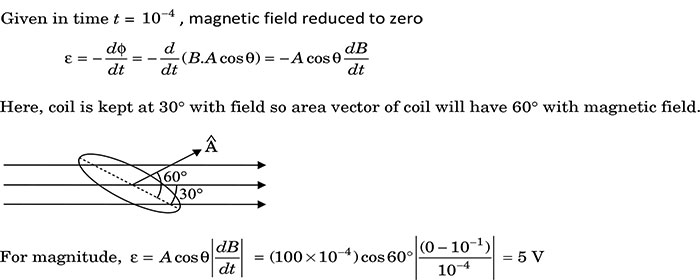## A 15 $\mathbf{\Omega }$ resistor, an 80 mH inductor and a capacitor of capacitance C are connected in series with a 50 Hz AC source. If the source voltage and current in the circuit are in phase, then the value of capacitance is

1. 100 $\mathrm{\mu F}$
2. 127 $\mathrm{\mu F}$
3. 142 $\mathrm{\mu F}$
4. 160 $\mathrm{\mu F}$

Ans. (b)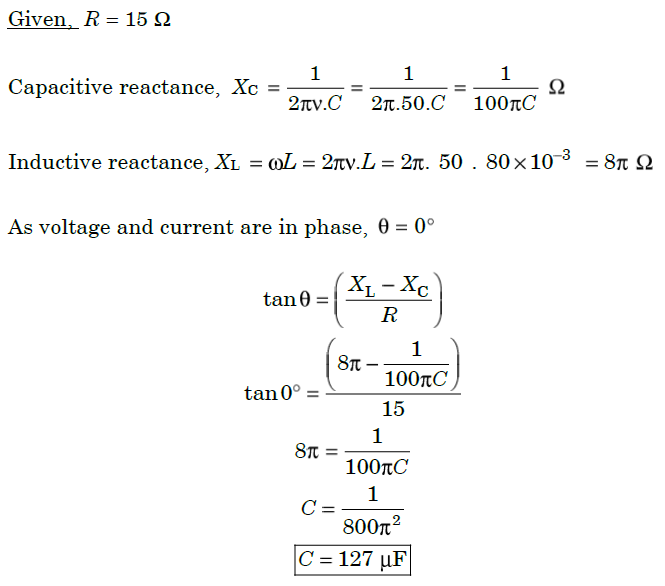## Four objects W, X, Y and Z, each with charge +q are held fixed at four points of a square of side d as shown in the figure. Objects X and Z are on the midpoints of the sides of the square. The electrostatic force exerted by object W on object X is F. Then the magnitude of the force exerted by object W on Z is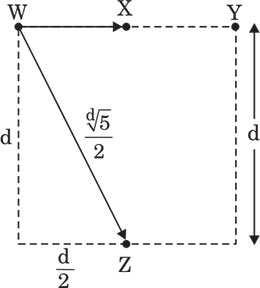1. $\frac{\mathrm{F}}{7}$
2. $\frac{\mathrm{F}}{5}$
3. $\frac{\mathrm{F}}{3}$
4. $\frac{\mathrm{F}}{2}$

Ans. (b)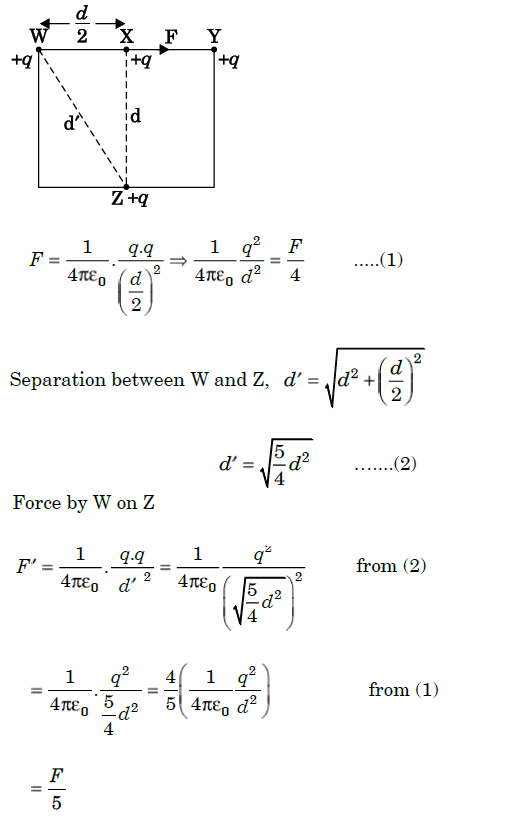## Two sources of equal emf are connected in series. This combination is, in turn connected to an external resistance R. The internal resistance of two sources are r1 and r2 (r2 > r1). If the potential difference across the source of internal resistance r2 is zero, then R equals to-

Ans. (b)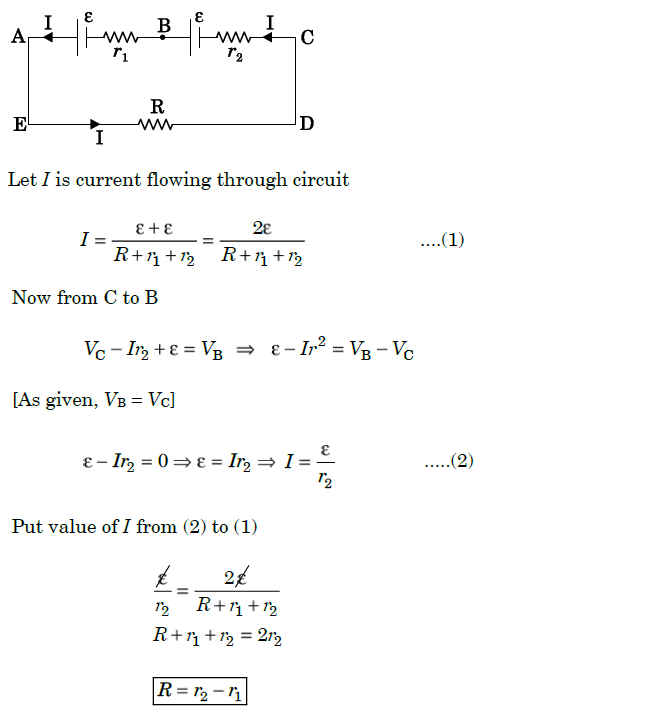## Which of the following statements is correct?

1. Magnetic field lines do not form closed loops.
2. Magnetic field lines start from north pole and end at south pole of a magnet.
3. The tangent at a point on a magnetic field line represents the direction of the magnetic field at that point.
4. Two magnetic field lines may intersect each other.

Ans. (c)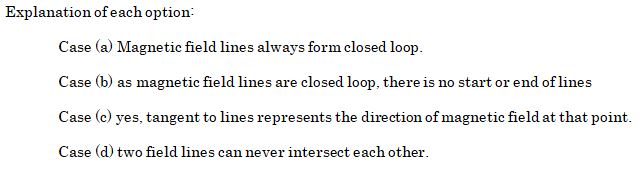## The equivalent resistance between A and B of the network shown in figure is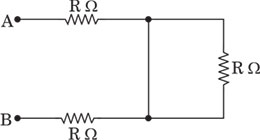1. 3R $\mathrm{\Omega }$
2. 2R $\mathrm{\Omega }$

Ans. (c)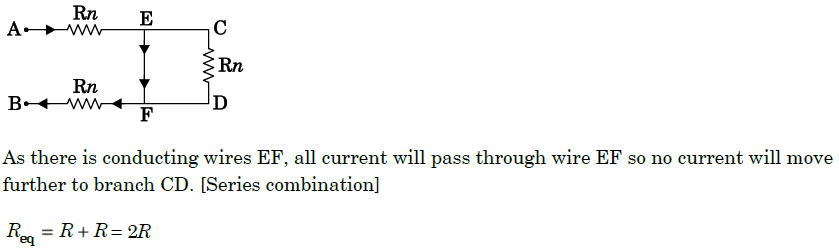## A bar magnet has magnetic dipole moment $\stackrel{\mathbf{\to }}{\mathbf{M}}$. Its initial position is parallel to the direction of uniform magnetic field $\stackrel{\mathbf{\to }}{\mathbf{B}}$. In this position, the magnitudes of torque and force acting on it respectively are –

1. 0 and MB
2. MB and MB
3. 0 and 0

Ans. (c)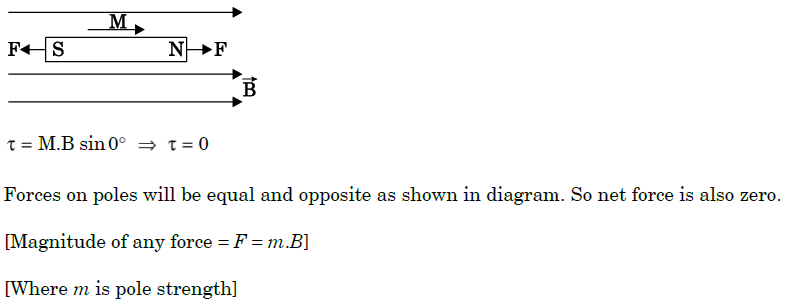1. 97.9 J
2. 102.1 J
3. 2.1 J
4. -97.9 J

Ans. (a)

## A 300 $\mathrm{\Omega }$ resistor and a capacitor of $\mathbf{\left(}\frac{\mathbf{25}}{\mathbf{\pi }}\mathbf{\right)}$$\mathrm{\mu }$F are connected in series to a 200 V - 50 Hz AC source. The current in the circuit is -

1. 0.1 A
2. 0.4 A
3. 0.6 A
4. 0.8 A

Ans. (b)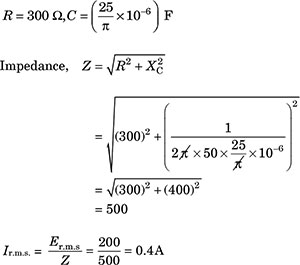## The core of a transformer is laminated to reduce the effect of:

1. flux leakage
2. copper loss
3. hysteresis loss
4. eddy current

Ans. (d)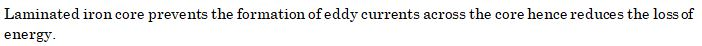Question No. 45 to 49 are Assertion (A) and Reason (R) type questions. Given below are the two statements labelled as Assertion (A) and Reason (R). Select the most appropriate answer from the options given below as:
1. Both a. and (R) are true and (R) is correct explanation of a.
2. Both a. and (R) are true and (R) is not correct explanation of a.
3. (A) is true, but (R) is false.
4. (A) is false and (R) is also false.

## Assertion (A): A negative charge in an electric field moves along the direction of the electric field.Reason (R): On a negative charge a force acts in the direction of the electric field

Ans. (d)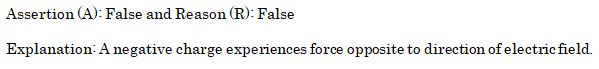## Assertion (A): The poles of a bar magnet cannot be separatedReason (R): Magnetic monopoles do not exist

Ans. (a)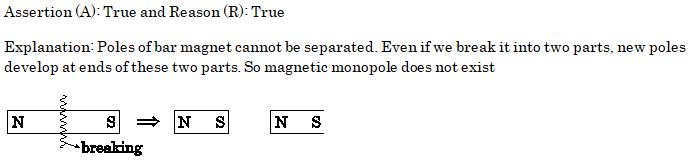## Assertion (A): When radius of a current carrying loop is doubled, its magnetic moment becomes four times.Reason (R): The magnetic moment of a current carrying loop is directly proportional to the area of the loop

Ans. (a)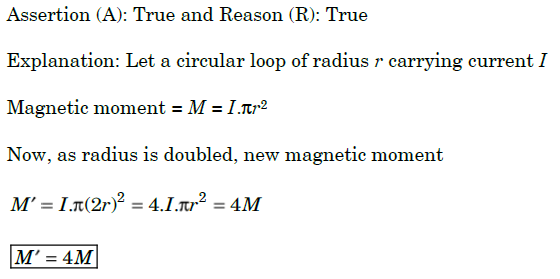## Assertion (A): Higher the range, lower is the resistance of an ammeter.Reason (R): To increase the range of an ammeter additional shunt is added in series to it.

Ans. (c)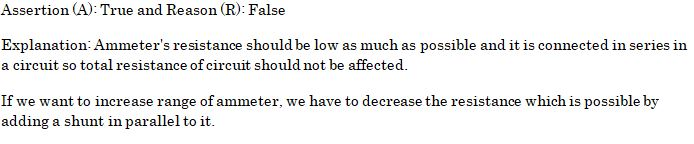## Assertion (A): A step-up transformer cannot be used as a step-down transformer.Reason (R): A transformer works only in one direction.

Ans. (d)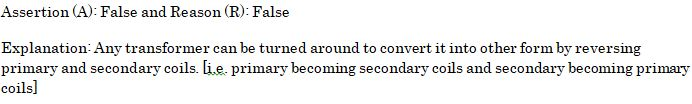## Equipotential at a large distance from a collection of charges whose total sum is not zero are-

1. spheres
2. planes
3. ellipsoids
4. paraboloids

Ans. (a)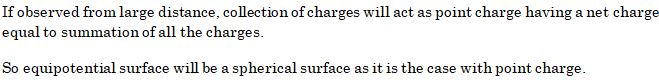## Four charges -q, -q, +q and +q are placed at the corners of a square of side 2L is shown in figure. The electric potential at point A midway between the two charges +q and +q is-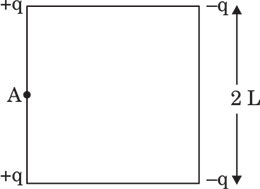1. zero

Ans. (a)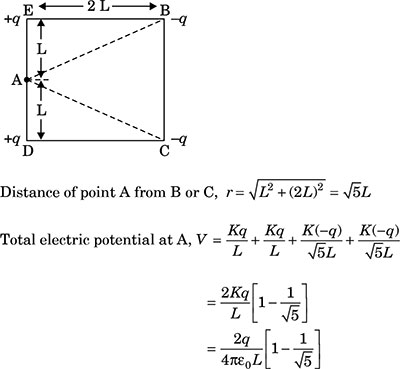### Case Study

An experiment was set up with the circuit diagram shown in figure. Given that R1 = 10 $\mathrm{\Omega }$, R2 = R3 = 5 $\mathrm{\Omega }$, r = 0 $\mathrm{\Omega }$ and E = 5 V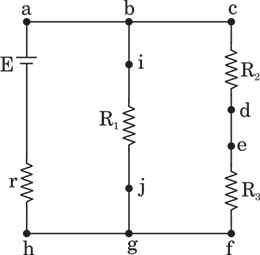## The points with the same potential are -

1. b, c, d
2. f, h, j
3. d, e, f
4. a, b, j

Ans. (b)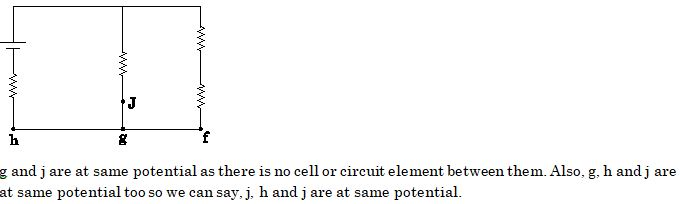## The current through branch bg is:

1. 1 A
2. $\frac{1}{3}\mathrm{A}$
3. $\frac{1}{2}\mathrm{A}$
4. $\frac{2}{3}\mathrm{A}$

Ans. (c)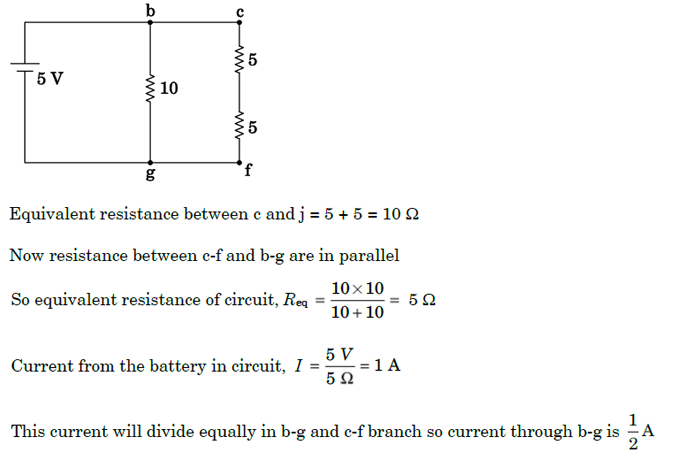## The power dissipated in R1 is:

1. 2 W
2. 2.5 W
3. 3 W
4. 4.5 W

Ans. (b)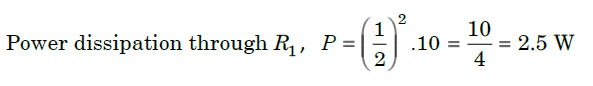## The potential difference across R3 is:

1. 1.5 V
2. 2 V
3. 2.5 V
4. 3 V

Ans. (c)